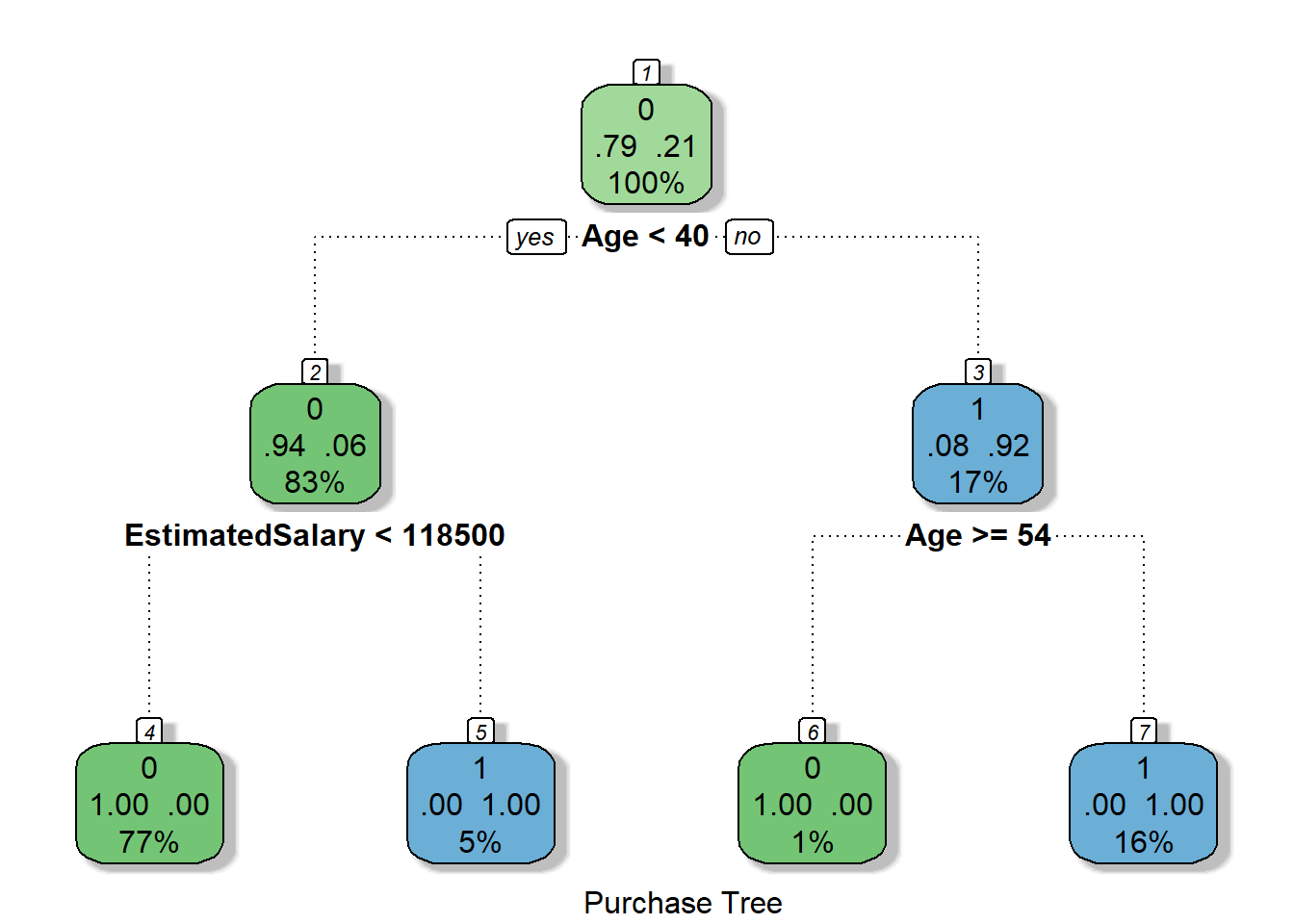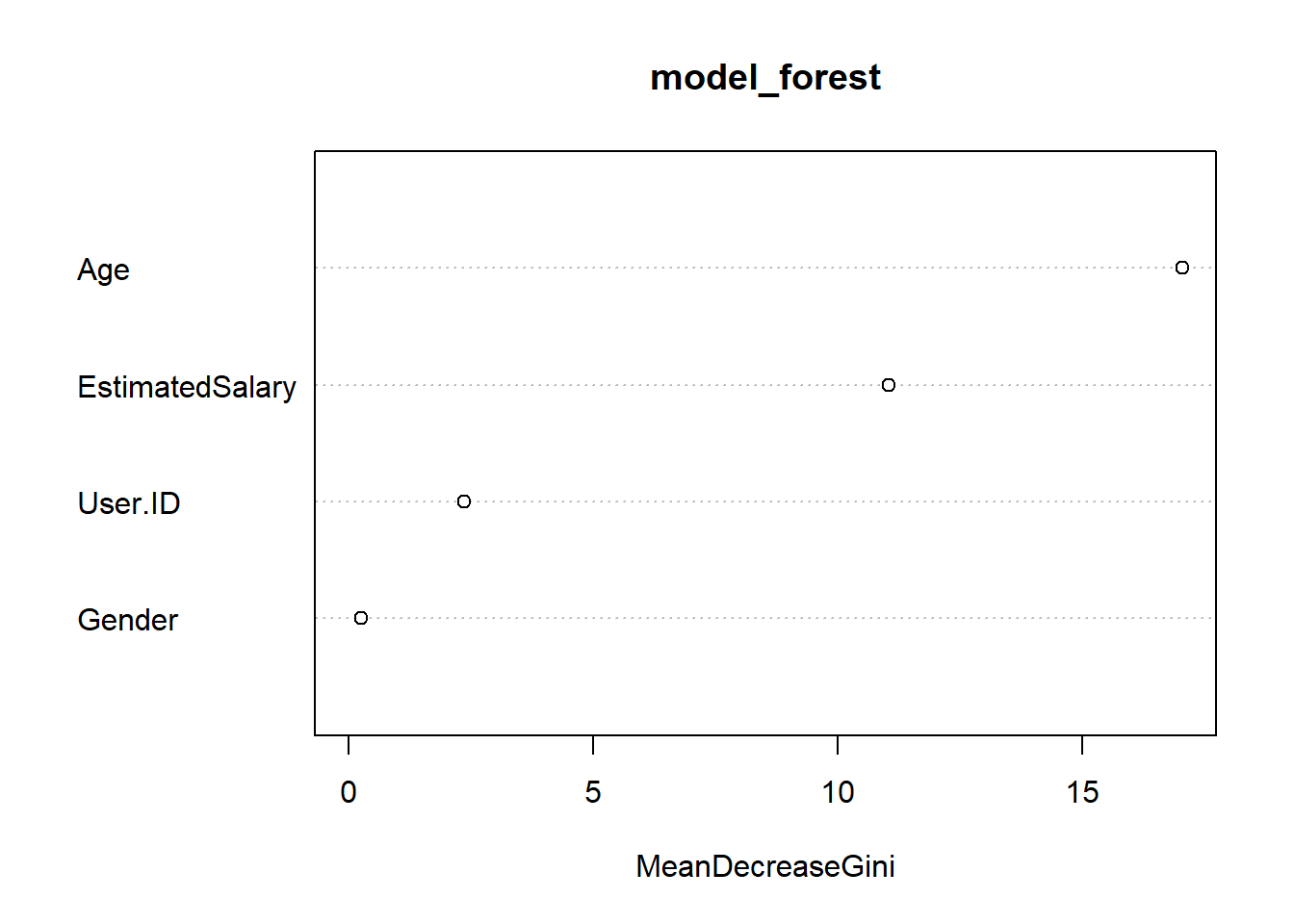# Butterfly Predictions

## Classification II

Random Forest Classifier is supervised classification/regression ensemble algorithm. Ensembled algorithms are those which combines more than one algorithm of same or different kind for classifying objects.

Random forest classifier creates a set of decision trees from randomly selected subset of training set. It then aggregates the votes from different decision trees to decide the final class of the test object.

In a nutshell if we have more trees in the forest robust the forest looks like. In the same way in the random forest algorithm the higher the number of trees in the forest gives the high accuracy results.

Use case:

In our set we are having data of recent purchases from any blog/ubscription, and now we would like to predict whether a new person will buy my subscription or not, data set is taken from super data science.

1. Decision Tree

In case of decision trees, only one tree structure will be formed where the root is decided based on information gain and you can understand whether the purchase will happen or not based on rules. See the below picture.

I plotted the tree rules for first 100 records in above set, and the results are below.

The numbers at the top of each node in the tree correspond to the branch numbers in the textual representation of the trees as generated by the default print () method.

The tree node has 4 values in all directions, North side represents the majority value of target node (in our case Purchased), West indicates the percentage values present with left condition and east for right condition percentage. South side value represents the percentage of values in that node, hence root node has maximum percentage.

This is our result with decision trees, 77% people with Age< 40 and Salary < 118K are not our target customers and 16% people with Age >54 turn out to be solid buyers.

2. Random Forest

If you are good with understanding decision tree, then you are not very far from learning what random forests are. There are two keywords here - random and forests.

Let us first understand what forest means. Random forest is a collection of many decision trees. Instead of relying on a single decision tree, you build many decision trees say 100 of them. And you know what a collection of trees is called - a forest.

Say our dataset has 50 rows and 5 columns. There are two levels of randomness in this algorithm:

At row level: Each of these decision trees in random forest gets a random sample of the training data (say 10%) i.e. each of these trees will be trained independently on 10 randomly chosen rows out of 50 rows of data. Keep in mind since each of these decision trees is getting trained on randomly chosen rows from the dataset i.e they are different from each other in terms of predictions.

At column level: The second level of randomness is introduced at the column level. Not all the columns are passed into training each of the decision trees. Say we want only 10% of columns to be sent to each tree. This means a randomly selected 3 columns will be sent to each tree.  So, for the first decision tree, may be column c1, c2 and c4 were chosen. The next DT will have c3, c4, C5 has chosen columns and so on.

The results from each of the tree are taken and the final output is declared accordingly.

Following code will show how we achieve these 2 in R and what will be the results for our set.

Code :

``````library(ggplot2)
library(rattle)``````
``````## Rattle: A free graphical interface for data science with R.
## Version 5.2.0 Copyright (c) 2006-2018 Togaware Pty Ltd.
## Type 'rattle()' to shake, rattle, and roll your data.``````
``````library(rpart)
library(randomForest)``````
``## randomForest 4.6-14``
``## Type rfNews() to see new features/changes/bug fixes.``
``````##
## Attaching package: 'randomForest'``````
``````## The following object is masked from 'package:rattle':
##
##     importance``````
``````## The following object is masked from 'package:ggplot2':
##
##     margin``````
``library(caret)``
``## Loading required package: lattice``

#### Step 2: Loading the data set and splitting into training and testing sets

``````Purchase<-read.csv("Social_Network_Ads.csv",header = TRUE)
p_train<-Purchase[1:75,]
p_test<-Purchase[75:100,]``````

#### Step 3 .Decision Tree model on train set

``````model_tree<-rpart(p_train\$Purchased~.,data=p_train,method="class",control=rpart.control(minsplit = 1,minbucket = 1,cp=0))
fancyRpartPlot(model_tree,sub = 'Purchase Tree',digits=-2)``````#### Step 4. Results and the confusion matrix for decision tree
``````p_test\$Purchased<-as.factor(p_test\$Purchased)
p_dt=predict(model_tree,newdata=p_test,type="class")
cm=confusionMatrix(data=p_dt,reference = p_test\$Purchased)
cm``````
``````## Confusion Matrix and Statistics
##
##           Reference
## Prediction  0  1
##          0 23  2
##          1  0  1
##
##                Accuracy : 0.9231
##                  95% CI : (0.7487, 0.9905)
##     No Information Rate : 0.8846
##     P-Value [Acc > NIR] : 0.4094
##
##                   Kappa : 0.4694
##  Mcnemar's Test P-Value : 0.4795
##
##             Sensitivity : 1.0000
##             Specificity : 0.3333
##          Pos Pred Value : 0.9200
##          Neg Pred Value : 1.0000
##              Prevalence : 0.8846
##          Detection Rate : 0.8846
##    Detection Prevalence : 0.9615
##       Balanced Accuracy : 0.6667
##
##        'Positive' Class : 0
## ``````

#### Step 4. Random forest model on same set

``````model_forest<-randomForest(as.factor(Purchase\$Purchased)~.,data =Purchase )
varImpPlot(model_forest)``````#### Step 6. Results and the confusion matrix for random forest
``````p_rf=predict(model_forest,newdata=p_test,type="class")
cm=confusionMatrix(data=p_rf,reference = p_test\$Purchased)
cm``````
``````## Confusion Matrix and Statistics
##
##           Reference
## Prediction  0  1
##          0 23  0
##          1  0  3
##
##                Accuracy : 1
##                  95% CI : (0.8677, 1)
##     No Information Rate : 0.8846
##     P-Value [Acc > NIR] : 0.04127
##
##                   Kappa : 1
##  Mcnemar's Test P-Value : NA
##
##             Sensitivity : 1.0000
##             Specificity : 1.0000
##          Pos Pred Value : 1.0000
##          Neg Pred Value : 1.0000
##              Prevalence : 0.8846
##          Detection Rate : 0.8846
##    Detection Prevalence : 0.8846
##       Balanced Accuracy : 1.0000
##
##        'Positive' Class : 0
## ``````

Conclusion : For our data set and first 100 rows , we get 92% accuracy with decsion tress and 100% accuracy with random forest.

Going forward we will be working on more requirement sets and how do we solve them.

Here is the next one in classification series

Bayes Thoerem and application

1.your article on data science is very good keep it up thank you for sharing.
1.2.3.4.